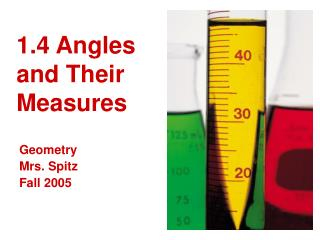DownloadDownload Presentation1.4 Angles and Their Measures

# 1.4 Angles and Their Measures

Download Presentation## 1.4 Angles and Their Measures

- - - - - - - - - - - - - - - - - - - - - - - - - - - E N D - - - - - - - - - - - - - - - - - - - - - - - - - - -
##### Presentation Transcript

1. 1.4 Angles and Their Measures Geometry Mrs. Spitz Fall 2005

2. Standard/Objectives: Standard: Students will understand geometric concepts and applications Benchmark: Use visualization, spatial reasoning, and geometric modeling to solve problems.

3. Standard/Objectives: Performance Standard: Solve problems involving complementary, supplementary and congruent angles. Objectives: • Use angle postulates • Classify angles as acute, right, obtuse, or straight.

4. Assignment: • pp. 29-31 #1-49 all

5. An angle consists of two different rays that have the same initial point. The rays are the sides of the angle. The initial point is the vertex of the angle. The angle that has sides AB and AC is denoted by BAC, CAB, A. The point A is the vertex of the angle. Using Angle Postulates

6. Name the angles in the figure: SOLUTION: There are three different angles. PQS or SQP SQR or RQS PQR or RQP Ex.1: Naming Angles You should not name any of these angles as Q because all three angles have Q as their vertex. The name Q would not distinguish one angle from the others.

7. Note: • The measure of A is denoted by mA. The measure of an angle can be approximated using a protractor, using units called degrees(°). For instance, BAC has a measure of 50°, which can be written as mBAC = 50°. B A C

8. Angles that have the same measure are called congruent angles. For instance, BAC and DEF each have a measure of 50°, so they are congruent. more . . . 50°

9. MEASURES ARE EQUAL mBAC = mDEF ANGLES ARE CONGRUENT BAC  DEF Note – Geometry doesn’t use equal signs like Algebra “is congruent to” “is equal to” Note that there is an m in front when you say equal to; whereas the congruency symbol  ; you would say congruent to. (no m’s in front of the angle symbols).

10. Consider a point A on one side of OB. The rays of the form OA can be matched one to one with the real numbers from 1-180. The measure of AOB is equal to the absolute value of the difference between the real numbers for OA and OB. Postulate 3: Protractor Postulate A O B

11. A point is in the interior of an angle if it is between points that lie on each side of the angle. A point is in the exterior of an angle if it is not on the angle or in its interior. Interior/Exterior

12. If P is in the interior of RST, then mRSP + mPST = mRST Postulate 4: Angle Addition Postulate

13. VISION. Each eye of a horse wearing blinkers has an angle of vision that measures 100°. The angle of vision that is seen by both eyes measures 60°. Find the angle of vision seen by the left eye alone. Ex. 2: Calculating Angle Measures

14. Classifying Angles • Angles are classified as acute, right, obtuse, and straight, according to their measures. Angles have measures greater than 0° and less than or equal to 180°.

15. Ex. 3: Classifying Angles in a Coordinate Plane • Plot the points L(-4,2), M(-1,-1), N(2,2), Q(4,-1), and P(2,-4). Then measure and classify the following angles as acute, right, obtuse, or straight. • LMN • LMP • NMQ • LMQ

16. Solution: • Begin by plotting the points. Then use a protractor to measure each angle.

17. Solution: • Begin by plotting the points. Then use a protractor to measure each angle. Two angles are adjacent angles if they share a common vertex and side, but have no common interior points.

18. Ex. 4: Drawing Adjacent Angles • Use a protractor to draw two adjacent acute angles RSP and PST so that RST is (a) acute and (b) obtuse.

19. Ex. 4: Drawing Adjacent Angles • Use a protractor to draw two adjacent acute angles RSP and PST so that RST is (a) acute and (b) obtuse.

20. Ex. 4: Drawing Adjacent Angles • Use a protractor to draw two adjacent acute angles RSP and PST so that RST is (a) acute and (b) obtuse. Solution:

21. Closure Question: • Describe how angles are classified. Angles are classified according to their measure. Those measuring less than 90° are acute. Those measuring 90° are right. Those measuring between 90° and 180° are obtuse, and those measuring exactly 180° are straight angles.